# Row_Number Function in MySQL

## Row_Number Function in MySQL with Examples

In this article, I am going to discuss Row_Number Function in MySQL with Examples. Please read our previous article where we discussed the OVER Clause in MySQL. At the end of this article, you will understand what exactly the ROW_NUMBER function is and when and how to use the Row Number function in MySQL with real-time examples.

##### ROW_NUMBER Function in MySQL:

The ROW_NUMBER function in MySQL is a window function or analytic function that is used when we want to return a unique sequential number starting from 1 for record in the result set. The ROW_NUMBER() function is a built-in function in MySQL that assigns a unique sequential integer number to each row starting from 1 within a partition of a result set. The row number always starts with 1 for the first row in each partition and then increases by 1 for the next row onwards in each partition.

The syntax to use the ROW_NUMBER function in MySQL is given below.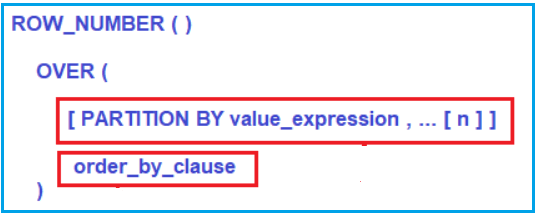###### Parameters:
1. PARTITION BY value_expression: This is optional. The PARTITION BY clause is used to partition the result set that is produced by the FROM clause and then the ROW_NUMBER function is applied to each partition. Here, the value_expression specifies the column name (s) using which the result set is going to be partitioned. As it is optional, if you did not specify the PARTITION BY clause, then the ROW_NUMBER function will treat all the rows of the query as a single partition.
2. Order_by_clause: This is optional. The ORDER BY clause is used to define the sequence in which each row is going to assign its unique ROW_NUMBER.
##### Examples to Understand ROW_NUMBER Function in MySQL

Let us see understand the ROW_NUMBER function in MySQL with some examples. We are going to use the following Employee table to understand the ROW_NUMBER function.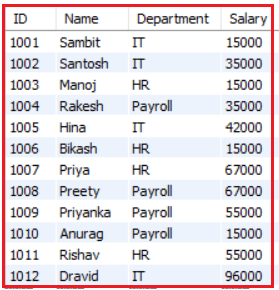Please use the below SQL Script to create the database and table as well as populate the Employees table with sample data.

```CREATE DATABASE EmployeeDB;
USE EmployeeDB;
CREATE TABLE Employees
(
ID INT PRIMARY KEY,
Name VARCHAR(50),
Department VARCHAR(50),
Salary int
);
INSERT INTO Employees Values (1001, 'Sambit', 'IT', 15000);
INSERT INTO Employees Values (1002, 'Santosh', 'IT', 35000);
INSERT INTO Employees Values (1003, 'Manoj', 'HR', 15000);
INSERT INTO Employees Values (1004, 'Rakesh', 'Payroll', 35000);
INSERT INTO Employees Values (1005, 'Hina', 'IT', 42000);
INSERT INTO Employees Values (1006, 'Bikash', 'HR', 15000);
INSERT INTO Employees Values (1007, 'Priya', 'HR', 67000);
INSERT INTO Employees Values (1008, 'Preety', 'Payroll', 67000);
INSERT INTO Employees Values (1009, 'Priyanka', 'Payroll', 55000);
INSERT INTO Employees Values (1010, 'Anurag', 'Payroll', 15000);
INSERT INTO Employees Values (1011, 'Rishav', 'HR', 55000);
INSERT INTO Employees Values (1012, 'Dravid', 'IT', 96000);
```
##### ROW_NUMBER Function without PARTITION BY Clause in MySQL:

Let us first see an example of the ROW NUMBER function without using the PARTITION BY Clause in MySQL. As we already discussed, if we did not specify the PARTITION BY Clause in MySQL, then the ROW_NUMBER function will treat the whole result set as a single partition. As a result, the ROW_NUMBER function will provide a consecutive sequence numbering for all the rows present in the result set based on the column(s) specified in the order by clause.

For better understanding, please have a look at the below example where we used the MySQL ROW_NUMBER function without using the PARTITION BY clause.

```SELECT Id, Name, Department, Salary,
ROW_NUMBER() OVER (ORDER BY Department) AS RowNumber
FROM Employees;```

Once you execute the above query, you will get the following output. If you observe the below output, then you can see that there is no partition and hence all the rows are assigned with consecutive sequence numbering starting from 1 to 12 based on the Department column.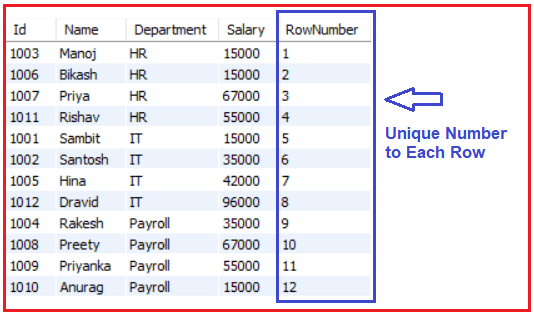If you did not specify the ORDER BY clause in the ROW_NUMBER then, it will give a unique number based on the default result set. For example, execute the below query which is without the ORDER BY clause.

##### MySQL Row_Number Function with PARTITION BY Clause:

Let us see an example of the MySQL ROW_NUMBER function with PARTITION BY Clause. When you specify the PARTITION BY Clause, then the data is partitioned based on the column you specify in the Partition by clause. Please have a look at the following code to understand this better. As you can see, here, we have specified Department in the Partition By clause and Name in the Order By clause.

```SELECT Id, Name, Department, Salary,
ROW_NUMBER() OVER
(
PARTITION BY Department
ORDER BY Name
) AS RowNumber
FROM Employees;
```

Once you execute the above query, you will get the following output. In our Employees table, we have three departments. So, the Partition by Clause will divide all the rows into three partitions (IT, HR, and Payroll). Then in each partition, the data is sorted based on the Name column. Finally, the ROW_NUMBER function gives a unique sequence number starting from 1 to each partition. As we have three partitions, each partition will start from 1 and which you can see in the below image.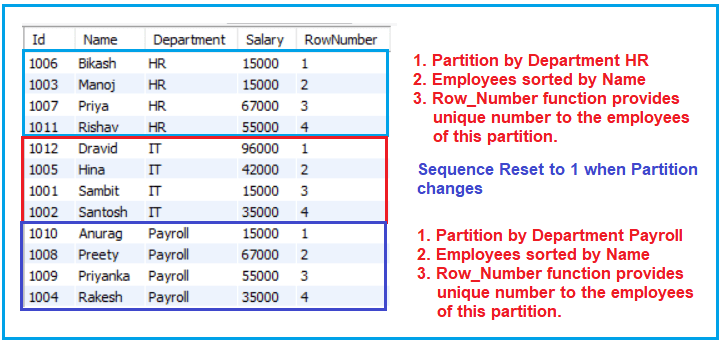##### Real-time Example of ROW_NUMBER function in MySQL:

Let us see one real-time example of the ROW NUMBER function in SQL Server. To understand this, we are going to use the following Employee table. Here, intentionally we have inserted some duplicate data into the Employee table.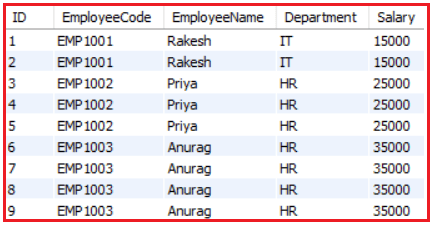Please use the below SQL Script to create the Employee table as well as populate the Employee table with the required sample data.

```CREATE TABLE Employee
(
ID INT PRIMARY KEY,
EmployeeCode VARCHAR(50),
EmployeeName VARCHAR(50),
Department VARCHAR(50),
Salary int
);

INSERT INTO Employee Values (1, 'EMP1001', 'Rakesh', 'IT', 15000);
INSERT INTO Employee Values (2, 'EMP1001', 'Rakesh', 'IT', 15000);
INSERT INTO Employee Values (3, 'EMP1002', 'Priya', 'HR', 25000);
INSERT INTO Employee Values (4, 'EMP1002', 'Priya', 'HR', 25000);
INSERT INTO Employee Values (5, 'EMP1002', 'Priya', 'HR', 25000);
INSERT INTO Employee Values (6, 'EMP1003', 'Anurag', 'HR', 35000);
INSERT INTO Employee Values (7, 'EMP1003', 'Anurag', 'HR', 35000);
INSERT INTO Employee Values (8, 'EMP1003', 'Anurag', 'HR', 35000);
INSERT INTO Employee Values (9, 'EMP1003', 'Anurag', 'HR', 35000);
```
##### Deleting Duplicate Records in MySQL using Row_Number Function:

Now we need to delete the duplicate records from the Employee table. Once we delete the duplicate records, then our Employee table should have the following three records.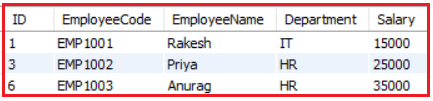The following SQL query will delete the duplicate records from the Employee table. Here, the PARTITION BY clause divides the result set into partitions based on the EmployeeCode column. That means it will create multiple partitions based on the Employee column value and give sequential numbers starting from 1 for each partition. Then the DELETE statements will delete the records from each partition where the RowNumber is greater than 1. It means it will keep only one record from each partition and delete the rest of all.

```WITH DeleteDuplicateEmployees
AS
(
SELECT Id, EmployeeCode, EmployeeName, Department, Salary,
ROW_NUMBER() OVER
(
PARTITION BY EmployeeCode
ORDER BY Id
) RowNumber
FROM Employee
)
DELETE FROM Employee USING Employee JOIN DeleteDuplicateEmployees
ON Employee.Id = DeleteDuplicateEmployees.Id
WHERE DeleteDuplicateEmployees.RowNumber > 1;
```

Here, we are using the common table expression (CTE) to return the duplicate rows by using the Row Number function, and then we are using the delete statement to remove the duplicate records from the Employee table.

Note: The point that you have to remember is, MySQL does not support CTE-based delete. Therefore, we have to join the original table with the CTE to achieve the same.

##### Finding top N rows of every group using Row Number function in MySQL

To understand how to find the top n rows of every group we are going to use the following Students table. As you can see, here, we categorized the students into three departments i.e. CSE, ETC, and CIVIL.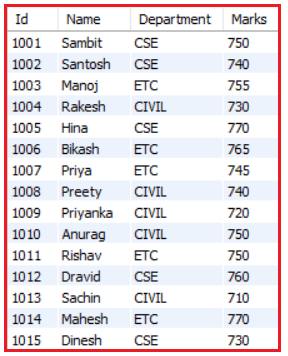Please use the following SQL Script to create the Students table as well as populate the Students table with the required data.

```CREATE TABLE Students
(
Id INT PRIMARY KEY,
Name VARCHAR(50),
Department VARCHAR(50),
Marks int
);

INSERT INTO Students Values (1001, 'Sambit', 'CSE', 750);
INSERT INTO Students Values (1002, 'Santosh', 'CSE', 740);
INSERT INTO Students Values (1003, 'Manoj', 'ETC', 755);
INSERT INTO Students Values (1004, 'Rakesh', 'CIVIL', 730);
INSERT INTO Students Values (1005, 'Hina', 'CSE', 770);
INSERT INTO Students Values (1006, 'Bikash', 'ETC', 765);
INSERT INTO Students Values (1007, 'Priya', 'ETC', 745);
INSERT INTO Students Values (1008, 'Preety', 'CIVIL', 740);
INSERT INTO Students Values (1009, 'Priyanka', 'CIVIL', 720);
INSERT INTO Students Values (1010, 'Anurag', 'CIVIL', 750);
INSERT INTO Students Values (1011, 'Rishav', 'ETC', 750);
INSERT INTO Students Values (1012, 'Dravid', 'CSE', 760);
INSERT INTO Students Values (1013, 'Sachin', 'CIVIL', 710);
INSERT INTO Students Values (1014, 'Mahesh', 'ETC', 770);
INSERT INTO Students Values (1015, 'Dinesh', 'CSE', 730);
```

We can use the ROW_NUMBER() function to find the top N rows for every group. For example, let us see how to find the top three students of each department based on the highest marks. The following SQL statement uses the ROW_NUMBER() to find the top three Students of Each Department based on the highest mark.

```WITH HighestMarkStudents
AS
(
SELECT Id, Name, Department, Marks,
ROW_NUMBER() OVER
(
PARTITION BY Department
ORDER BY Marks DESC
) RowNumber
FROM Students
)
SELECT Id, Name, Department, Marks
FROM HighestMarkStudents
WHERE RowNumber <= 3;
```

Once you execute the above query, you will get the following desired output.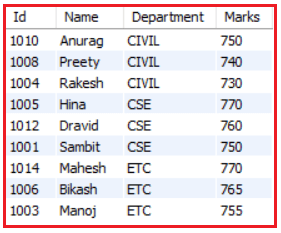##### Pagination using ROW_NUMBER() function in MySQL

As the MySQL ROW_NUMBER() assigns a unique integer number to each row in the result, we can use it for pagination. For example, we need to display a list of Students with 5 students per page. To get all the Students for the second page, we need to use the following SQL query.

```SELECT * FROM
(
SELECT Id, Name, Department, Marks,
ROW_NUMBER() OVER  (ORDER BY Id) AS RowNumber
FROM Students
) temp
WHERE RowNumber BETWEEN 6 AND 10;
```

Once you execute the above query, you will get the following desired output.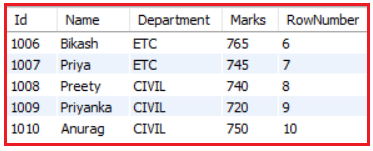In the next article, I am going to discuss the RANK function in MySQL with examples. Here, in this article, I try to explain the need and use of the ROW_NUMBER function in MySQL with Examples. I hope you enjoy this ROW NUMBER function in the MySQL article.